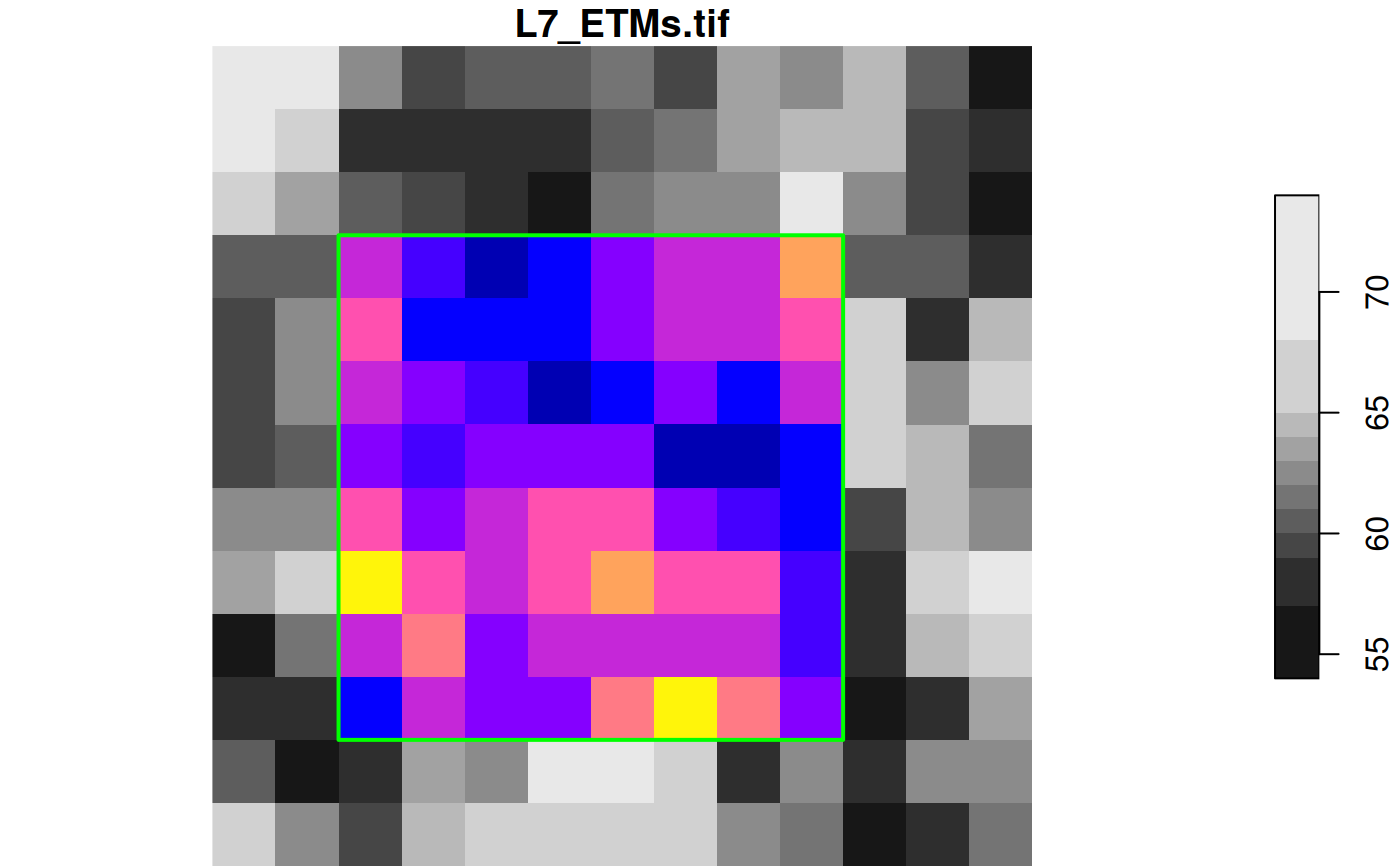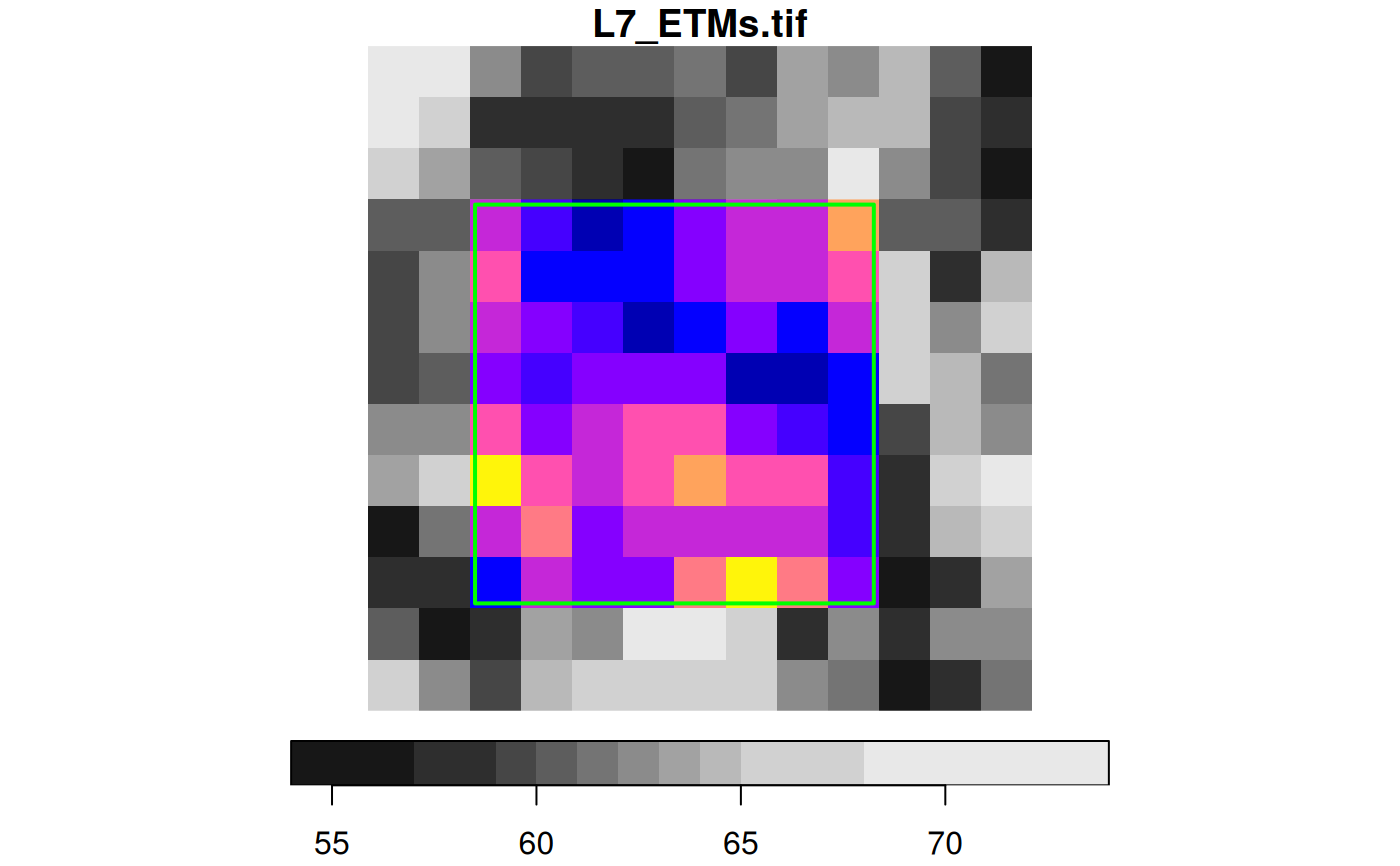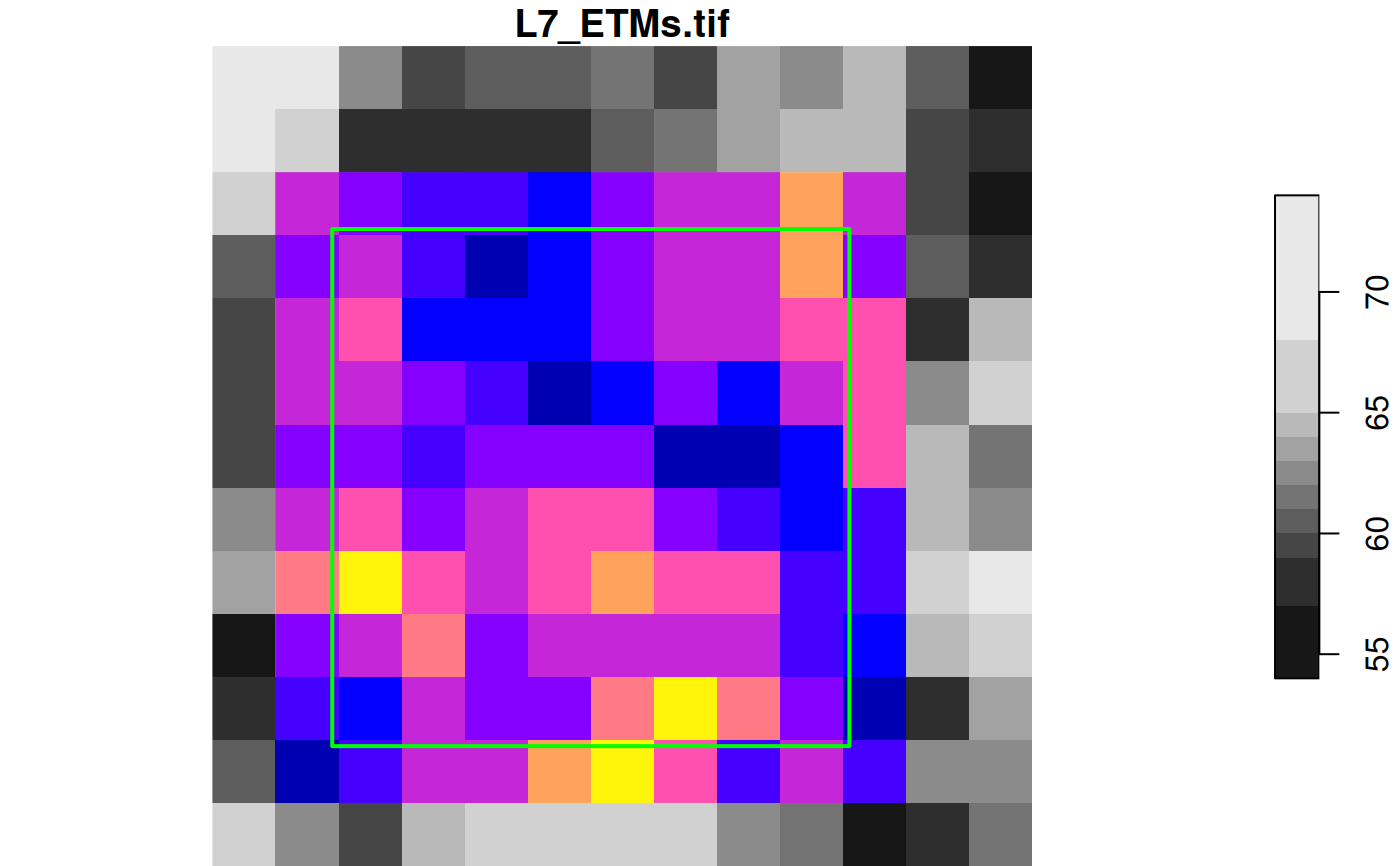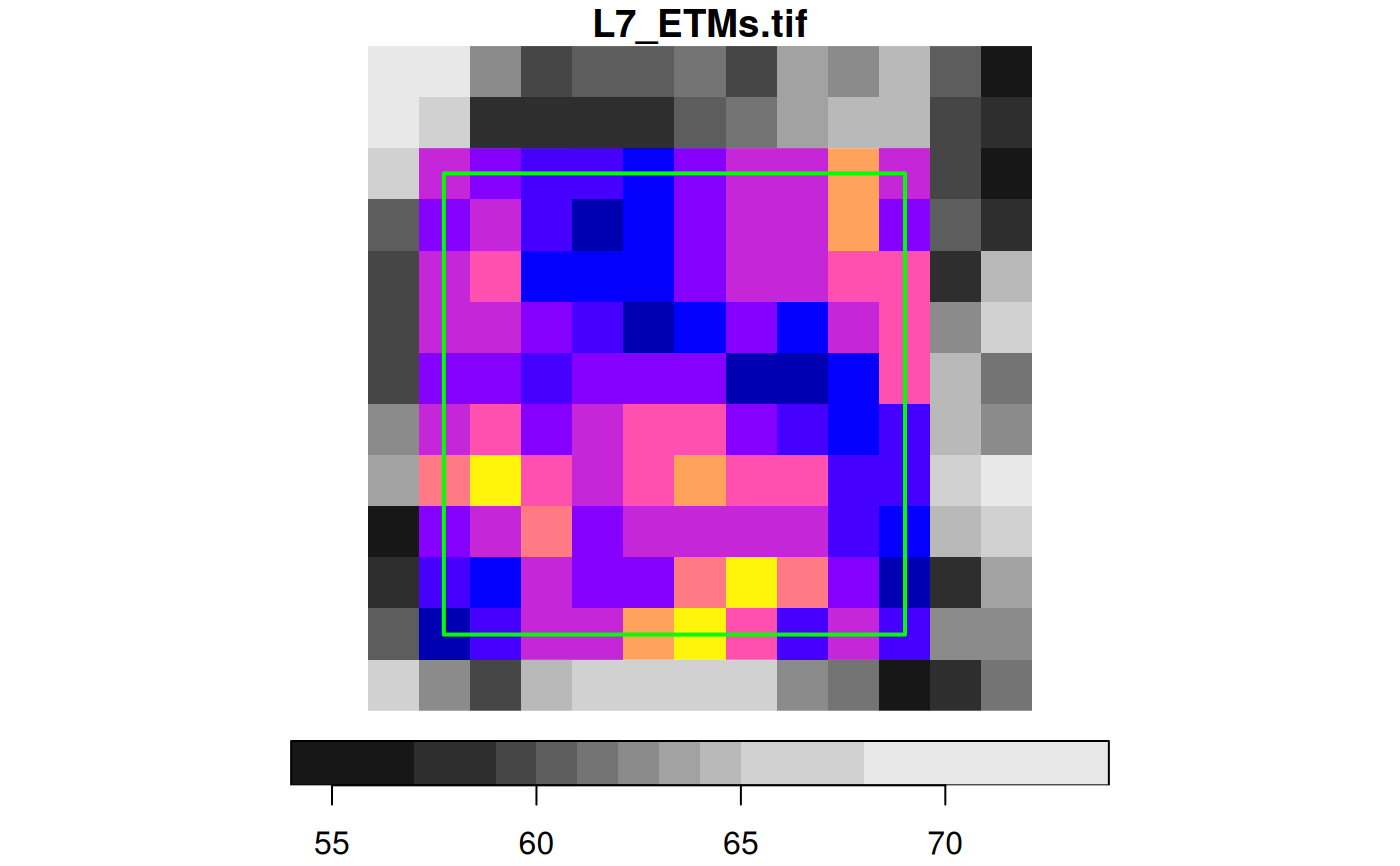crop a stars object

# S3 method for stars_proxy
st_crop(
x,
y,
...,
crop = TRUE,
epsilon = sqrt(.Machine$double.eps), collect = TRUE ) # S3 method for stars st_crop( x, y, ..., crop = TRUE, epsilon = sqrt(.Machine$double.eps),
as_points = all(st_dimension(y) == 2, na.rm = TRUE)
)

## Arguments

x

object of class stars

y

object of class sf, sfc or bbox; see Details below.

...

ignored

crop

logical; if TRUE, the spatial extent of the returned object is cropped to still cover obj, if FALSE, the extent remains the same but cells outside y are given NA values.

epsilon

numeric; factor to shrink the bounding box of y towards its center before cropping.

collect

logical; if TRUE, repeat cropping on stars object, i.e. after data has been read

as_points

logical; only relevant if y is of class sf or sfc: if FALSE, treat x as a set of points, else as a set of small polygons. Default: TRUE if y is two-dimensional, else FALSE; see Details

## Details

for raster x, st_crop selects cells that intersect with y. For intersection, are raster cells interpreted as points or as small polygons? If y is of class stars, x raster cells are interpreted as points; if y is of class bbox, x cells are interpreted as cells (small polygons). Otherwise, if as_points is not given, cells are interpreted as points if y has a two-dimensional geometry.

## Examples

l7 = read_stars(system.file("tif/L7_ETMs.tif", package = "stars"))
d = st_dimensions(l7)

# area around cells 3:10 (x) and 4:11 (y):
offset = c(d[["x"]]$offset, d[["y"]]$offset)
res = c(d[["x"]]$delta, d[["y"]]$delta)
bb = st_bbox(c(xmin = offset + 2 * res,
ymin = offset + 11 * res,
xmax = offset + 10 * res,
ymax = offset +  3 * res), crs = st_crs(l7))
l7[bb]
#> stars object with 3 dimensions and 1 attribute
#> attribute(s):
#>              Min. 1st Qu. Median     Mean 3rd Qu. Max.
#> L7_ETMs.tif    17      43   55.5 56.36979      65  111
#> dimension(s):
#>      from to  offset delta                     refsys point values x/y
#> x       3 10  288776  28.5 SIRGAS 2000 / UTM zone 25S FALSE   NULL [x]
#> y       4 11 9120761 -28.5 SIRGAS 2000 / UTM zone 25S FALSE   NULL [y]
#> band    1  6      NA    NA                         NA    NA   NULL
# equivalent:
st_crop(l7, bb)
#> stars object with 3 dimensions and 1 attribute
#> attribute(s):
#>              Min. 1st Qu. Median     Mean 3rd Qu. Max.
#> L7_ETMs.tif    17      43   55.5 56.36979      65  111
#> dimension(s):
#>      from to  offset delta                     refsys point values x/y
#> x       3 10  288776  28.5 SIRGAS 2000 / UTM zone 25S FALSE   NULL [x]
#> y       4 11 9120761 -28.5 SIRGAS 2000 / UTM zone 25S FALSE   NULL [y]
#> band    1  6      NA    NA                         NA    NA   NULL

plot(l7[,1:13,1:13,1], reset = FALSE)
image(l7[bb,,,1], add = TRUE, col = sf.colors())
plot(st_as_sfc(bb), add = TRUE, border = 'green', lwd = 2)# slightly smaller bbox:
bb = st_bbox(c(xmin = offset + 2.1 * res,
ymin = offset + 10.9 * res,
xmax = offset +  9.9 * res,
ymax = offset +  3.1 * res), crs = st_crs(l7))
l7[bb]
#> stars object with 3 dimensions and 1 attribute
#> attribute(s):
#>              Min. 1st Qu. Median     Mean 3rd Qu. Max.
#> L7_ETMs.tif    17      43   55.5 56.36979      65  111
#> dimension(s):
#>      from to  offset delta                     refsys point values x/y
#> x       3 10  288776  28.5 SIRGAS 2000 / UTM zone 25S FALSE   NULL [x]
#> y       4 11 9120761 -28.5 SIRGAS 2000 / UTM zone 25S FALSE   NULL [y]
#> band    1  6      NA    NA                         NA    NA   NULL

plot(l7[,1:13,1:13,1], reset = FALSE)
image(l7[bb,,,1], add = TRUE, col = sf.colors())
plot(st_as_sfc(bb), add = TRUE, border = 'green', lwd = 2)# slightly larger bbox:
bb = st_bbox(c(xmin = offset + 1.9 * res,
ymin = offset + 11.1 * res,
xmax = offset + 10.1 * res,
ymax = offset +  2.9 * res), crs = st_crs(l7))
l7[bb]
#> stars object with 3 dimensions and 1 attribute
#> attribute(s):
#>              Min. 1st Qu. Median     Mean 3rd Qu. Max.
#> L7_ETMs.tif    17      43     55 56.11167      66  112
#> dimension(s):
#>      from to  offset delta                     refsys point values x/y
#> x       2 11  288776  28.5 SIRGAS 2000 / UTM zone 25S FALSE   NULL [x]
#> y       3 12 9120761 -28.5 SIRGAS 2000 / UTM zone 25S FALSE   NULL [y]
#> band    1  6      NA    NA                         NA    NA   NULL

plot(l7[,1:13,1:13,1], reset = FALSE)
image(l7[bb,,,1], add = TRUE, col = sf.colors())
plot(st_as_sfc(bb), add = TRUE, border = 'green', lwd = 2)# half a cell size larger bbox:
bb = st_bbox(c(xmin = offset + 1.49 * res,
ymin = offset + 11.51 * res,
xmax = offset + 10.51 * res,
ymax = offset +  2.49 * res), crs = st_crs(l7))
l7[bb]
#> stars object with 3 dimensions and 1 attribute
#> attribute(s):
#>              Min. 1st Qu. Median     Mean 3rd Qu. Max.
#> L7_ETMs.tif    17      43     55 56.11167      66  112
#> dimension(s):
#>      from to  offset delta                     refsys point values x/y
#> x       2 11  288776  28.5 SIRGAS 2000 / UTM zone 25S FALSE   NULL [x]
#> y       3 12 9120761 -28.5 SIRGAS 2000 / UTM zone 25S FALSE   NULL [y]
#> band    1  6      NA    NA                         NA    NA   NULL

plot(l7[,1:13,1:13,1], reset = FALSE)
image(l7[bb,,,1], add = TRUE, col = sf.colors())
plot(st_as_sfc(bb), add = TRUE, border = 'green', lwd = 2)